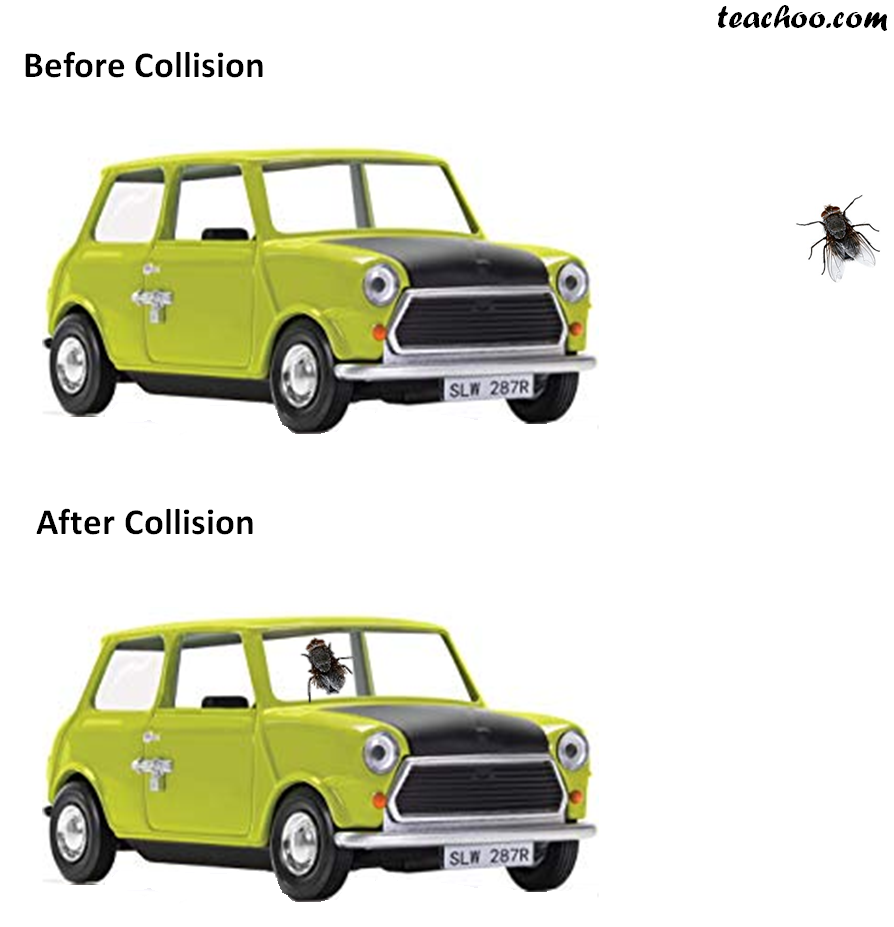NCERT Questions

Class 9
Chapter 9 Class 9 - Force and Laws Of Motion

## Akhtar, Kiran and Rahul were riding in a motorcar that was moving with a high velocity on an expressway when an insect hit the windshield and got stuck on the windscreen. Akhtar and Kiran started pondering over the situation. Kiran suggested that the insect suffered a greater change in momentum as compared to the change in momentum of the motorcar (because the change in the velocity of the insect was much more than that of the motorcar). Akhtar said that since the motorcar was moving with a larger velocity, it exerted a larger force on the insect. And as a result the insect died. Rahul while putting an entirely new explanation said that both the motorcar and the insect experienced the same force and a change in their momentum. Comment on these suggestions.Let’s look what all 3 people said

• Kiran – Due to greater change in momentum of insect, as  compared to car. And the insect died
• Akhtar - Since motorcar was moving with a larger velocity, it exerted a larger force on the insect. And the insect died.
• Rahul - Both motorcar and insect experienced the same force and change in their momentum.

According to law of conservation of momentum,

Total Momentum before collision = Total momentum after collision

When the insect hits the car,

it experiences larger change in momentum

(Because Initial momentum will be less because it's mass is less, and final momentum will be much more because mass of car + insect is a lot)

Hence, Kiran’s observation is correct.

If the car was moving slowly, the insect might not have died.

Since the car was moving with high velocity, it exerted higher force on the insect.

So, Akhtar's observation is correc t.

Now, Rahul said that both motorcar and insect experienced the same force and change in their momentum.

Rahul is partially correct   Because

• According to third law of motion - For every action, there is an equal and opposite reaction.
So, the force exerted by car on insect will be same as force exerted by insect on car.
• But, Change in momentum of insect will be much higher than change in momentum of car

GREY BOX FOR BETTER UNDERSTANDING

Let's take an example

Mass of car = 1000 kg

Velocity of car = 100 m/s

Mass of insect = 1 kg

Velocity of insect = -1 m/s  (Negative as insect is moving in opposite direction of car)

When they collide,

insect and car move together

Finding Initial and Final Momentum

Initial Momentum = Mass of car × Velocity of car + Mass of insect × Velocity of insect

= 1000 × 100 + 1 × (-1)

= 100000 - 1

= 99999

Final Momentum = Mass of (car + insect) × Velocity of (car + insect)

= (1000 + 1) × v

= 1001v

Now,

Initial Momentum = Final Momentum

99999 = 1001v

1001v = 99999

v = 99999/1001

v = 99.89

Thus,

Change in momentum of car = Final momentum of (car + insect)  - Initial Momentum of car

= 1001 × 99.89 - 1000 × 100

= 99999 - 100000

= -1

Change in momentum of insect = Final momentum of (car + insect)  - Initial Momentum of insect

= 1001 × 99.89 - 1 × (-1)

= 99999 + 1

= 100000

So, we can see that change of momentum of insect is much more than change of momentum of car

Learn in your speed, with individual attention - Teachoo Maths 1-on-1 Class